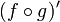# Composite of two functions

## Definition

Suppose$f,g$ are two functions. The composite function$f \circ g$ is defined as the function:$f \circ g = x \mapsto f(g(x))$

Note that the function written at the right end of the composition is the function performed first, and the function written at the left end of the composition is the function performed next. We say that composition of functions is done right-to-left.

## Relation with various operations

Below, we discuss how a particular operation done for functions can be done for a composite of two functions:

Operation Verbal description How it's done
Graph$f \circ g$ We are given the graphs of$f$ and$g$ (without necessarily having algebraic, numerical, or verbal descriptions of the functions) and we need a geometric method to sketch the graph of$f \circ g$ graphing the composite of two functions
Obtain explicit expression for$f \circ g$ We are given explicit algebraic expressions for$f$ and$g$ and need an explicit algebraic expression for$f \circ g$. simple case: finding the composite of two functions by plugging in expressions
case of piecewise functions: finding the composite of two piecewise functions
Find limit of$f \circ g$ at a point We have techniques for finding limits for both functions, we need a technique for finding the limit of the composite. composition theorem for continuous functions
Differentiate$f \circ g$. We have expressions for the derivatives$f'$ and$g'$, we need an expression for$(f \circ g)'$. chain rule for derivatives:$(f \circ g)' = (f' \circ g) \cdot g'$.
Integrate$f \circ g$. We want to integrate$f \circ g$ in terms of integration of simpler functions. We can try integration by u-substitution or integration by parts.